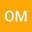Another way to prove the finite difference for a monomial is equal to the degree factorial
•• Oliver Mansbach
Oliver Mansbach

Corresponding Author:revilo.ojm@gmail.com

Author Profile## Abstract

In the past, Isaac Newton had proved that using the finite difference method on a monomial will give the coefficient times the degree factorial. He had proven it using calculus. The finite difference method is used to find the degree of any polynomial, when only given a couple of outputs. This is very useful when given a pattern of numbers and when you must determine the initial polynomial. In my proof, I will prove this without using calculus. Instead, I will use manipulations of summation and pascal's triangle. Isaac Newton and I were both trying to prove the following equation:
(See first line of proof)